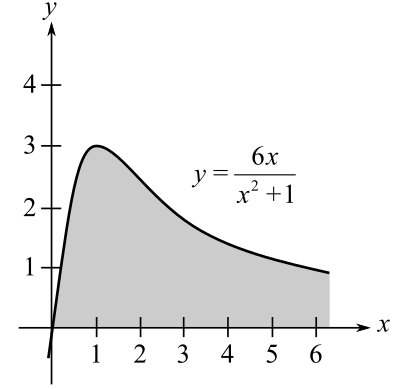Chapter 6.4, Problem 25E### Calculus: An Applied Approach (Min...

10th Edition
Ron Larson
ISBN: 9781305860919

#### Solutions

Chapter
Section### Calculus: An Applied Approach (Min...

10th Edition
Ron Larson
ISBN: 9781305860919
Textbook Problem
1 views

# Area of a Region In Exercises 21-26, find the area of the unbounded shaded region. y = 6 x x 2 + 1To determine

To calculate: The area of the unbounded shaded region for the function y=6xx2+1,Explanation

Given Information:

The provided function is, y=6xx2+1 and the graph is:

Formula used:

The area between two graphs:

If f(x)g(x) for all x in [a,b] (so that the graph of f doesn’t move below that of g), then the area of the region between the graphs of f and g and between x=a and x=b is given by:

A=ab[f(x)g(x)dx]

From definition of improper integral.

af(x)dx=limbabf(x)dx

Also, the expression for the integration of an exponential is as follows:

eaxdx=eaxa+C; where a0.

Calculation:

Here, the two functions are f(x)=6xx2+1 and g(x)=0 and the graph is going from 0 to .

So, the area of the region between the graphs of f(x)=6xx2+1 and g(x)=0 and between x=0 and x= is given by:

A=0[(6xx2+10)dx]=06xx2+1dx

Use the property of improper integral and simplify as:

### Still sussing out bartleby?

Check out a sample textbook solution.

See a sample solution

#### The Solution to Your Study Problems

Bartleby provides explanations to thousands of textbook problems written by our experts, many with advanced degrees!

Get Started

#### Find more solutions based on key concepts